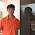## Thursday, February 7, 2013

### JVM Parameter SurvivorRatio

This post is very small but useful, I published this coz during my search I faced quite trouble to get the meaning of SurvivorRatio JVM parameters which is been used to segregate the memory between Eden space and Survivor spaces.
In JVM you can segregate the Young generation from total heap using these parameters e.g. -XX:NewSize=400m -XX:MaxNewSize=400m or -XX:NewRatio=3
In ideal condition 40% vs 60% ratio will be good between Young Generation and Old Generation respectively; alternatively it will depend on what type of application you have deployed on JVM and based on GC.log output values can be tuned further..
To defined explicit memory area for young generation used the below settings. For example if you have 1gb heap size define using this parameter –Xms1024m –Xmx1024m or –Xms1g –Xmx1g then give this “XX:NewSize=400m -XX:MaxNewSize=400m” to define young generation area.
Now there in one more important configuration which you need to consider while segregating young generation that’s called ‘SurvivorRatio’.
SurvivorRatio been used to further split the memory areas in Young Generation and controls the size of the two survivor spaces, young generation contains three sub area 1) Eden Space 2) Survivor One 3) Survivor Two
Eden Space has two Survivor spaces. One survivor space is empty at any given time. These Survivor Spaces serves as the destination of the next copying collection of any living objects in Eden and the other survivor space. If survivor spaces are too small copying collection overflows directly into the tenured generation.

During my search for survivor ratio I found various blogs, each blogs were saying different things, some of them saying, if you set the SurvivorRatio=6 it will set the ratio between each survivor space and eden to be 1:6, other were saying “each survivor space will be 1/8 the young generation” etc but I didn’t get any such blog which gave me crystal clear formula which will help to drive the Survivor space size.

I decided to do some POC and build a formula for that, so that Survivor ratio can be calculated easily.

Here is the formula which I have derived –

Formula to calculate S1 or S2 -
Total Young Generation / (SurvivorRatio+2)*2
E.g. If you have Young generation 400 Mb, SurvivorRatio set to 8 below will be output-

400 / (8+2) *2 = 20 MB

Similarly here is the formula to derived Eden space –

Formula for Eden Space –

(SR +1) * Young Gen / (SR +2)

E.g. if you have Young generation 400 Mb, SurvivorRatio set to 8 below will be output-

8+1*400/8+2 = 3600/10 = 360 MB

Result of few values of SurvivorRatio which I have tested in Test Environment -

 Young Generation S1 S2 Eden SurvivorRatio 400 33.31 33.31 333.38 4 400 25 25 350 6 400 20 20 360 8

Note:
As per above result, if you decrease the SurvivorRatio value then you will get more Survivor Space, if you Increase SurvivorRatio you will get less Survivor Space.

1.hi, the calculation for survivor seems to be wrong. I've tried something and check my calculation in wily and matches.

ex: Young gen - 2048 MB. Survivor ratio :2
formula used - (Young Gen)/Survivor ratio+2. So 2048/4 = 512 MB for each survivor space. Eventually eden space is 1024 MB and each survivor space is 512 MB.

2.very useful graphic..nicely written.

3.If SurvivorRatio=6 then the ratio of (One SurvivorSpace : Eden) = (1:6). So two Survivor will take (2 part), and Eden has 6 parts. So total 8 parts. So if i have young gen 40 MB, Eden is (6/8 * 40) and survivor is (1/8 * 40).

if SurvivorRatio=7. Then total parts are (7 + 1 + 1 = 9).
Eden is (7/9 * 40) and survivor is (1/9 * 40)